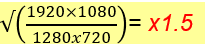# Different performances of zoom functions

Last update: September, 2020

This article describes how the below 3 zoom functions work and shows sample charts of relationship between image capture size and zoom ratio.

#### Optical zoom

Enlarges and reduces a subject image by zooming the lens in and out while shooting.
Fixed lens models do not have optical zoom function.

#### Digital Zoom

Zooms in by enlarging the cropped image by way of software.
Image degradation occurs due to the loss of image resolution.

#### Extra optical zoom

Shows the image cropped from the highest resolution image when "Image capture size" is set to lower than the model's highest resolution.
An image without degradation can be displayed.
Extra optical zoom is not available with the maximum resolutions.
The zoom ratio that works with extra optical zoom without switching to digital zoom varies depending on the "Image capture size" setting.

<Sample of FHD Model with Optical Zoom(x2.5)>
When "Image capture size" is set to 640×360, an enlarged image without degradation can be displayed with its zoom ratio value from x2.5 up to x7.5 as the 640×360 image([B] in the below) cropped from the largest size of 1920×1080 image ([A] in the below) is displayed as a zoomed in image. (Below are sample images of WV-U-1132)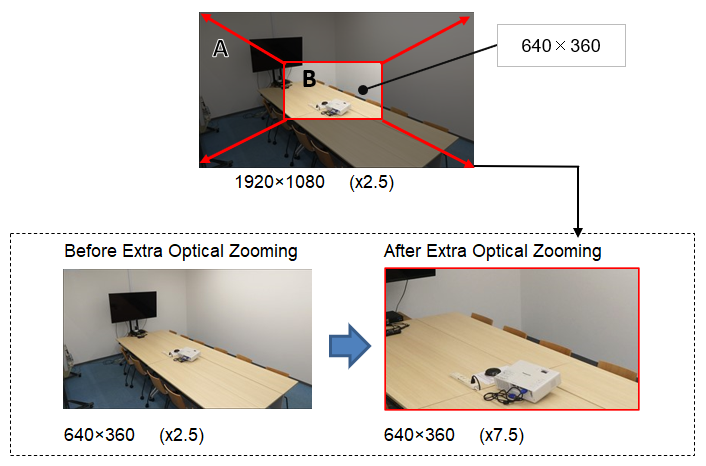#### Specifications of varifocal models on zooming

The below charts show the zoom ratio of 4MP models (WV-U1142, WV-U1542L, WV-U2142L, WV-U2542L) and FHD models (WV-U1132, WV-U1532L, WV-U2132L, WV-U2532L) as examples.
Specific value of extra optical zoom ratio for each model can be calculated with the formula in "Note".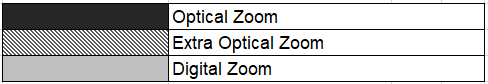4MP Models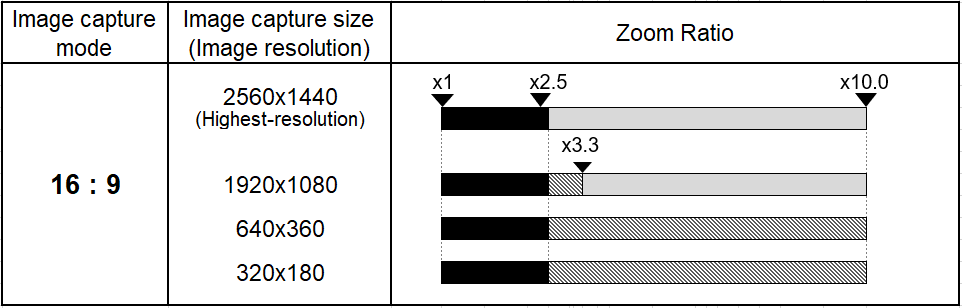FHD Models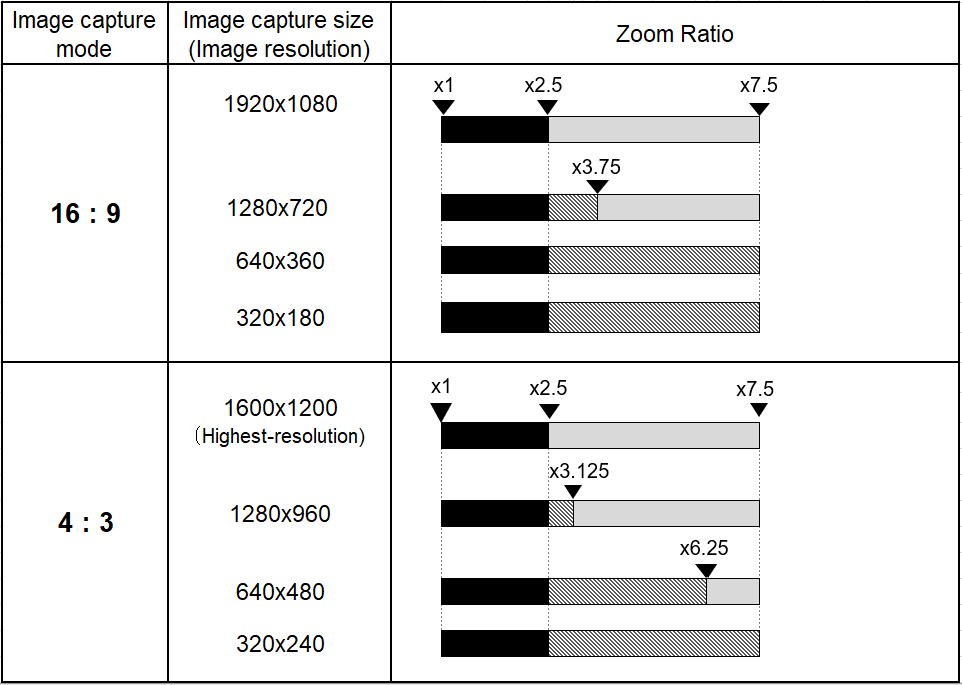Note:
- As optical zoom ratio and selectable image capture size vary depending on the model, refer to the manuals or setting screen of the model in use.
- As for the maximum zoom ratio for extra optical zoom, you can calculate with the below calculation formula.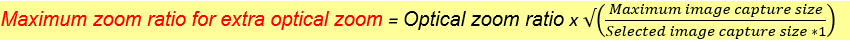*1  The image capture size selected for the stream in camera setting

[Sample calculation]
The maximum zoom ratio for extra optical zoom when "Image capture mode=16：9"
and "Image capture size=1280x720" in the above chart can be calculated as below.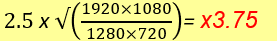#### Specifications of Fixed Lens Models on Zooming

The below charts show the zoom ratio of 4MP models (WV-U2140L, WV-U2540L) and FHD models (WV-U1130, WV-U2130L, WV-U2530L) as examples.
Specific value of extra optical zoom ratio for each model can be calculated with the formula in "Note".4MP Models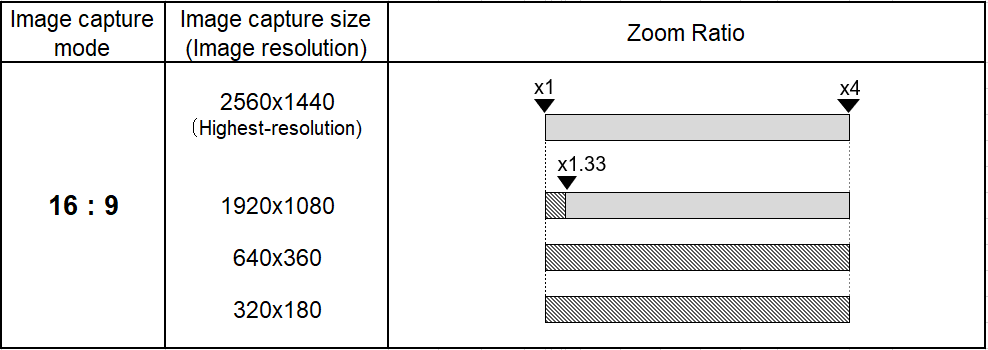FHD Models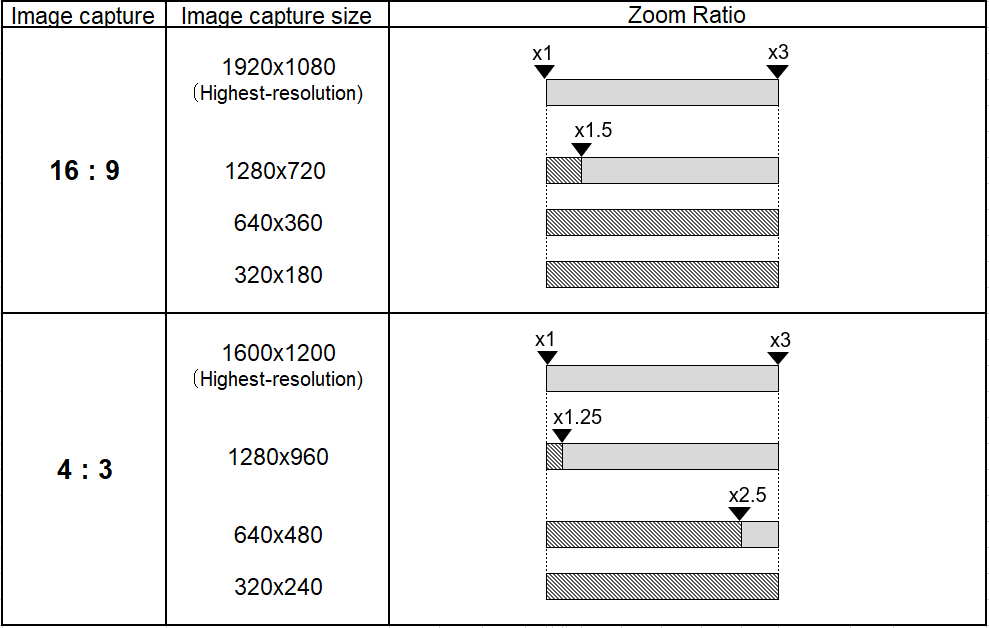Note:
- As optical zoom ratio and selectable image capture size vary depending on the model, refer to the manuals or setting screen of the model in use.
- As for the maximum zoom ratio for extra optical zoom, you can calculate with the below calculation formula.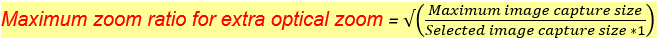*1  The image capture size selected for the stream in camera setting

[Sample calculation]
The maximum zoom ratio for extra optical zoom when "aspect ratio=16：9"
and "Image capture size=1280x720" can be calculated as below.